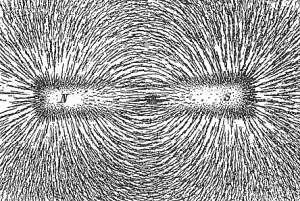# The Four Fundamental Forces of Nature

1. Home
2. /
3. The Four Fundamental Forces...

Physicists inform us there are four fundamental forces in nature.

Many scientists believe fundamental forces will one day be explained by means of a single, unified field theory. So far no one has come up with a viable mathematical package.

## Four Fundamental Forces

Currently, scientists speak of the four fundamental forces as the gravitational force, the weak force, the electromagnetic force, and the strong force. Each force has a sphere of influence.

## Gravitational Force

Gravity is the weakest force. But it is far reaching, essentially infinite. It is associated with the mass of objects (example, M1 and M2) and the distance between them (d) according to the relation,

F(grav) = G(M1)(M2)/d2

G is the gravitational constant. The gravitational force exchange particle is the hypothetical graviton.

## The Weak Force

The weak force is short ranged. It is predominates in radioactive decay and neutrino interactions. It is the force within the nucleus. It is involved in the unification or dissociation of a neutron and its component parts—an electron, a proton, and a neutrino,

no → p+ + e + ν

Weak bosons are the key particles of exchange.

## The Electromagnetic Force

Electromagnetic force relates to the presence and interactions between charged particles. Electromagnetic force covers electrostatic and magnetic phenomena. The field of quantum electrodynamics (classical or relativistic), is used to explain interactions at the subatomic level.Electromagnetic force has an infinite range of interaction. It is considerably stronger than the gravitational and weak forces. The photon is the key particle of exchange for electromagnetic force.

## The Strong Nuclear Force

The strong nuclear force operates over a very short range, but it is the strongest of the forces. It holds quarks together to form nuclear particles, and keeps the nucleons (protons and neutrons) together within the nucleus. This interaction involves the back and forth exchange of pi mesons between nuclear particles.

Note: You might also enjoy Lotus Effect: Vibrations and Superhydrophobic Behavior

References:

### 2 thoughts on “The Four Fundamental Forces of Nature”

• […] The Weak Force – short-range force within the nucleus of the atom that predominates in neutrino interactions and radioactive decay. (2) […]